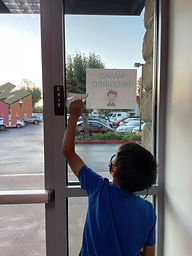## Ms. Caitlyn

### Target 1​

###### Lesson Type:

New

Number Operation

:

Number System

Use understandings of positive and negative number order to determine the value of a given space on a number line.

###### 1:

Understand that a number line is a spatial representation of quantities.

###### 2:

Understand that numbers have an unchanging order, so even if a number is not written on the number line (it still has a space).

###### 3:

Indicate an integer on the number line.

6th

###### Vocabulary:

Activities:

• Students completed an exploration where they added and subtracted integers.
• Students identified patterns between adding and subtracting positive and negative numbers.
• Students found task cards throughout the center and solved them.
• Students graphed the answers to their task cards on a number line.### Home Exploration

###### Guiding Questions:## Absent Students:

### Target 2

:

###### 1:

Complete a number sequence in both ascending and descending order.

###### 2:

Be able to start a number sequence from any given number.

5th

###### Vocabulary:

Sequence, Positive, Negative

Activities:

• Students sorted Post-Its that were out of order into their corresponding sequences.### Home Exploration

###### Guiding Questions:### Target 3

:

###### 1:

Study a given arithmetic sequence to determine what rule explains the sequence (i.e., 2-4-6-8-10…each number is the sequence gets bigger by 2).

###### 3:

Define multiplication and division patterns.

###### 4:

Understand that a pattern can be described by stating the rule that it is following.

6th

###### Vocabulary:

Sequence, Pattern

Activities:

• Students played a matching game, where they matched the rule to the sequence.### Home Exploration